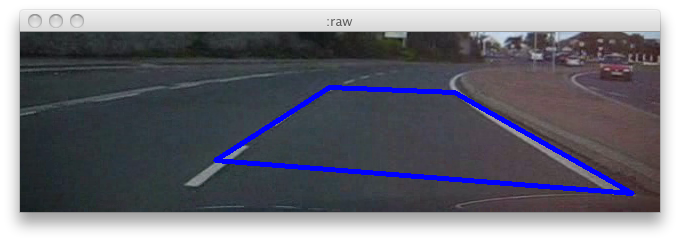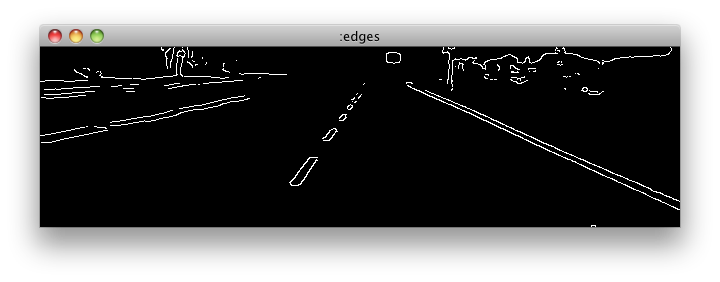Lane Detection using Clojure and OpenCV

Recently I had a simple requirement, locate a colored marker in a video feed and broadcast its location via UDP. My initial solution was to do the detection using OpenCV and broadcast it using Clojure. Once done I decided to split the single locate call into individual OpenCV calls so I can have more control over the process from Clojure side without recompiling the C library. One thing led to another and it got to a point where it can do all the things Processing OpenCV wrapper can and more, so I decided to release it, you can grab it here.

Following snippet demonstrates a quick and dirty way to do lane detection.(ns lane-detection

(defn detect-edges [i]
(--> (convert-color i :bgr-gray)
(smooth :gaussian 7 7 0 0)
(canny 90 90 3)))

For every frame, we convert it to gray scale, smooth it, and mark the edges on the scene using the Canny algorithm. —> in the code is not a typo, none of the calls in the library modify the original image, instead they all return a brand new image which needs to be released when done, —> works just like the -> in Clojure with the only difference being, it will call release for each intermediate image. After edge detection we end up with the following,(defn points [i]
(->> (hough-lines i :probabilistic 1 (/ Math/PI 180) 15 40 50)
flatten
(partition 2)))

(defn polygon [points]
(let [dist (fn [[x1 y1] [x2 y2]]
(Math/sqrt (+ (Math/pow (- x2 x1) 2) (Math/pow (- y2 y1) 2))))]
[(first (sort-by #(dist [300 0] %) points))
(first (sort-by #(dist [450 10] %) points))
(first (sort-by #(dist [640 80] %) points))
(first (sort-by #(dist [200 80] %) points))]))

After edge detection, our next step is to find the lines in the image, for that we use hough-lines which finds lines in a binary image using a Hough transform. When using the :probabilistic method it returns a sequence of point pairs by flattening it and then partitioning it by 2, we end up with a sequence of points. Then polygon function picks 4 points from this sequence that is closest to the top center, top right, bottom center, bottom right of the image. (This obviously only works when you are on the right lane and there isn't a white car in front of you.)

(let [k 0.80
vals (ref nil)]

(defn moving-filter [x f]
(+ (* k f) (* (- 1.0 k) x)))

(defn filter [pts]
(dosync (ref-set vals (if (not (nil? @vals))
(partition 2 (map moving-filter
(flatten pts)
(flatten @vals)))
pts)))))

(defn process-frame [f w h]
(let [roi (copy-region f 0 50 w (- h 50))
edges (detect-edges roi)
points (points edges)
lane  (map #(let [[x y] %] [x (+ y 50)]) (filter (polygon points)))]
(poly-line f lane java.awt.Color/blue 4)
(release [roi edges])))

process-frame puts all of the above together, for each frame first we remove the horizon from the image, detect edges and find points that make up the lane, run those points through a moving average filter to reduce flickring and finally draw the lane on the original frame.

(let [capture (capture-from-file "StayingInLane_MPEG4.avi")
frame-count (get-capture-property capture :frame-count)
width (get-capture-property capture :frame-width)
height (get-capture-property capture :frame-height)]

(dotimes [_ frame-count]
(let [frame (query-frame capture)]
(process-frame frame width height)
(view :raw frame)